# Kepler's problem by H-J method

Kepler's problem is basically a problem related to central force. For simplicity we take inverse square force between nucleus of charge 'Ze' and electron of charge 'e'.

One of the advantages of using the H-J method to solve Kepler's problem is that it provides a systematic way to obtain a complete set of solutions for the motion of a particle under the influence of an inverse square law force, such as gravity.

The H-J method allows us to separate the variables of the Hamilton-Jacobi equation for Kepler's problem, which is a partial differential equation that describes the motion of a particle under the influence of a central force. By separating the variables, we can reduce the partial differential equation to a set of ordinary differential equations that are easier to solve. These ordinary differential equations provide a complete set of solutions for the motion of the particle, which can be used to determine the trajectory, velocity, and position of the particle at any point in time.

Another advantage of using the H-J method to solve Kepler's problem is that it provides a deeper understanding of the underlying physics of the problem. The H-J method is based on the principle of least action, which states that the path taken by a particle is the one that minimizes the action, or the integral of the Lagrangian over time. By using the H-J method, we can derive the Lagrangian for Kepler's problem, which describes the motion of the particle in terms of its position and velocity. This allows us to better understand the physical principles that govern the motion of the particle and how they relate to the solutions obtained using the H-J method. In this case, Hamiltonian can be expressed asWhere pr and pθ are the components of momentum for r and θ. Since the system is conservative and H doesn't involve time explicitly so,

H = α1 = E (say )...........2

As there is no time occurring in the expression of it so the generating function S in this case is Hamilton's characteristics function W. Thus, we can write

pi = ∂W/∂qi

pr = ∂W/∂r and pθ = ∂W/∂θ .............3

With the help of equation 3, equation 1 can be written as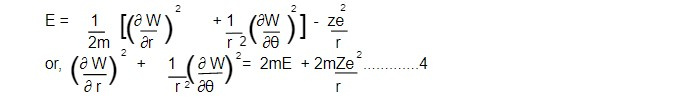Using the method of variable separation, we have

W = Wr + Wθ ......5

Using eq 5 in eq 4, we getAgain, we know that the angular momentum is always conserved in the central force problem i.e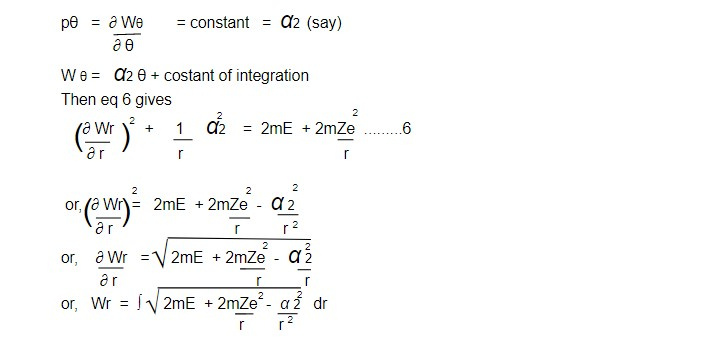Hence complete solution becomes

W = Wθ + Wr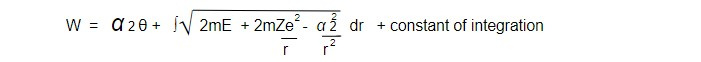θ1 = β + t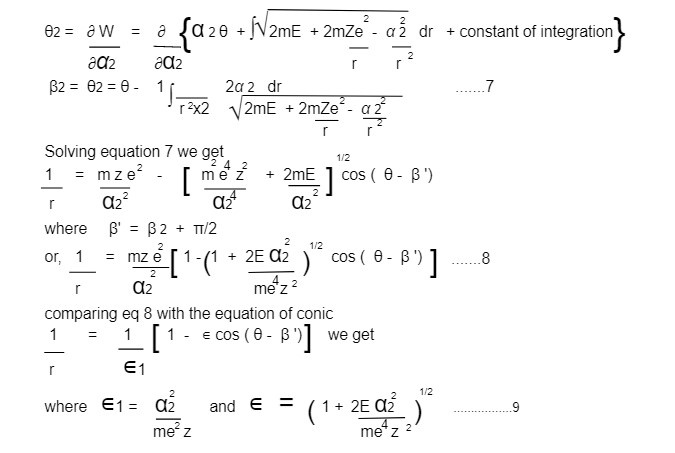For E<0, ∊ <1, the path of the particle is ellipse.

For E =0, ∊ = 1, the path of the particle is parabola.

For E>0, ∊ >1, the path of the particle is hyperbola.

Kepler's problem in action angle variables

Kepler's problem can also be solved using the action-angle variables, which are a set of variables that describe the motion of a system in terms of its conserved quantities, such as energy and angular momentum. In the case of Kepler's problem, the conserved quantities are the energy, the magnitude of the angular momentum, and the projection of the angular momentum onto the z-axis, which is perpendicular to the plane of motion.

The action-angle variables for Kepler's problem are known as the Keplerian action and the Keplerian angle. The Keplerian action is proportional to the area swept out by the particle in its orbit over a fixed period of time, while the Keplerian angle is the angle between the position of the particle and a fixed reference direction, such as the direction of the pericenter.

To solve Kepler's problem using the action-angle variables, we first need to derive the equations of motion in terms of the action-angle variables. This can be done using Hamilton's equations, which relate the time derivatives of the action-angle variables to the partial derivatives of the Hamiltonian, which describes the energy of the system.

The solution of Kepler's problem using the action-angle variables is highly efficient, because the equations of motion are simplified in terms of the conserved quantities, which makes the problem easier to solve than using other variables. The action-angle variables provide a simple and intuitive way to describe the motion of the particle, and they are commonly used in the field of celestial mechanics to model the orbits of planets, asteroids, and comets around the Sun, or the orbits of moons around planets.

To exhibit all of the solution, we need to consider the motion in 3D space. In terms of spherical polar coordinate (r, θ, 𝜙), the Hamiltonian of the system is given bywhere V(r) = - k/r ...........2

If the variables in the corresponding H-J equation are separable, then W must have the form

W = W(r) + Wθ(θ) + W𝜙(𝜙)

with p𝜙 = ∂W𝜙 /∂𝜙 = α𝜙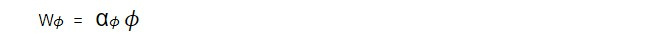pr = ∂Wr /∂r , pθ = ∂Wθ/∂θ

Thus the H-J equation reduce to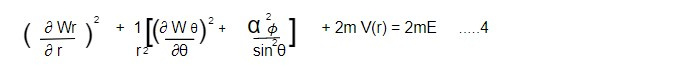The H-J equation then confirms that the quantity in the square bracket must be a constant.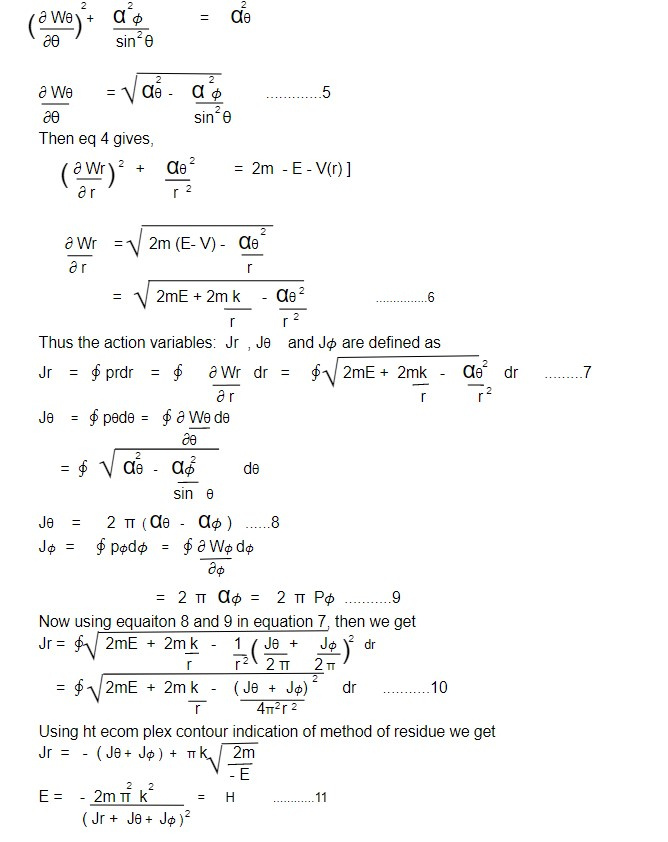All the three of the action variables appear only in the form Jr + J +J𝜙. Hence all the frequencies are equal. The motion is completely degenerate. Thus the one frequency for the motion is given by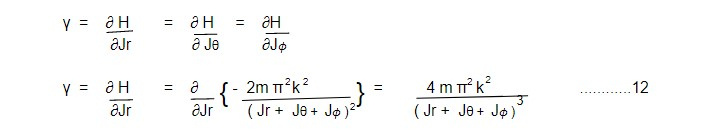if we evaluate the sum of J's in terms of the energy (E) from equations 11, the frequency is given by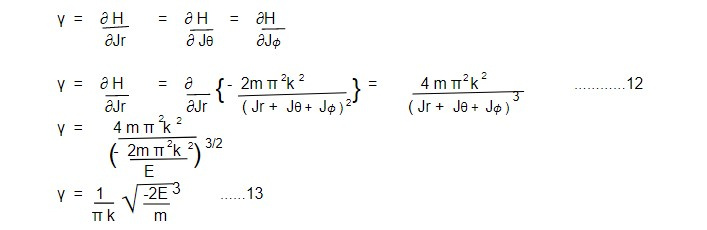And the time period of the orbit is given by

𝜏 = 1/ γ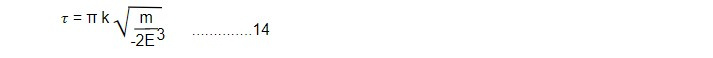Thus the equation 13 and 14 gives the frequency and time period for the motion in spherical polar coordinate system.

This note is taken from Classical Mechanics, MSC physics, Nepal.

This note is a part of the Physics Repository.